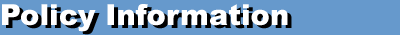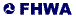An update of the manual is available! - HPMS Field Manual

 << Previous Contents

## HPMS Field Manual

### Appendix N: Procedures for Estimating Highway Capacity

#### Multilane Highway Capacity

##### Application

The following procedures are applied to rural and urban multilane highways with the following characteristics:

• Partial or no access control (Data Item 55 = 2 or 3) with a Divided Cross-Section (see above under "Freeways") OR Full Access Control and Undivided Cross-Section; and

• 4 or more through lanes and two-way operation (Data Item 34 >= 4 and Data Item 27 = 2 ) OR 2-3 through lanes and one-way operation (Data Item 34 = 2 or 3 and Data Item 27 = 1.

##### Procedure

Step 1: Calculate Free Flow Speed (FFS)

The first step in the procedure is to estimate free flow speed (FFS) of the facility. HCM Equation 21-1 is applied directly:

 FFS = BFFS - fLW - fLC- fM - fA (6)

Where:

 BFFS = base free flow speed fLW = adjustment factor for lane width fLC = adjustment factor for lateral clearance fM = adjustment factor for median type fA = adjustment factor for access points

Base Free Flow Speed

Base Free Flow Speed is based on the coded speed limit (Data Item 80) and guidance from the HCM 2000. To be consistent with the HCM 2000 methodology, the BFFS is not allowed to go below 40 mph or above 70 mph. This conflicts with guidance in the HCM 2000 which states that the methodology is valid for free flow speeds between 45 mph and 60 mph. However, the HCM 2000 methodology is geared to estimating performance characteristics, not capacity; for the purpose of capacity, these restrictions were relaxed.

 BFFS = 40 mph, for posted speed limits < 40 mph (7) = SpeedLimit + 7, for posted speed limits 40-45 mph = SpeedLimit + 5, for posted speed limits >= 50 mph

Where: SpeedLimit is the value for Data Item 80.

Adjustment Factor for Lane Width (fLW)

The values from HCM Exhibit 21-4 are used and directly based on the values of Data Item 54:

Lane Width Reduction in FFS (mph; fLW)
12 ft. 0.0
11 ft. 1.9
<=10 ft. 6.6

Adjustment Factor for Lateral Clearance (fLC)

The values from HCM Exhibit 21-5 are used and based on computing Total Lateral Clearance (TLC) using right and left shoulder widths. Lateral Clearance Left (LCL) is computed for divided highways only (Data Item 57 >= 4 or Data Item 56 = 1 or Data Item 56 = 2). In all other cases, such as if a continuous two-way left turn lane exists (Data Item 88 = 2) or the facility is undivided, then LCL is set to 6. (The median type adjustment factor accounts for the fact that the roadway is undivided.) Facilities with one-way traffic operation are considered divided highways since there is no opposing flow to interfere with traffic. For one-way facilities, left shoulder width is not coded. Therefore, a value of 6 is assumed for one-way facilities.

 TLC = LCR+ LCL (8)

Where:

 LCR = Data Item 59, maximum value of 6 LCL = Data Item 60, maximum value of 6

Once TLC is computed, the values in HCM Exhibit 21-5 are used. Linear interpolation is used for intermediate values:

##### Table 4. Adjustment for Lateral Clearance
4-Lane Two-Way Highways and
2-Lane One-way Highways
6+Lane Two-Way Highways and
3+Lane One-Way Highways
Total LateralClearance Reduction in FFS Total Lateral Clearance Reduction in FFS
12 0.0 12 0.0
10 0.4 10 0.4
8 0.9 8 0.9
6 1.3 6 1.3
4 1.8 4 1.7
2 3.6 2 2.8
0 5.4 0 3.9

Adjustment Factor for Median Type (fM)

The values from HCM Exhibit 21-6 are used and based on whether the facility is divided or undivided. (See definition under Lateral Clearance Adjustment).

Highway Type Reduction in FFS (mph; fM)
Undivided 1.6
Divided (including TWLTLs-
Two-Way Left Turning Lanes)
0.0

Adjustment Factor for Access Points (fA)

The number of access points per mile is based directly on the values of the number of at-grade intersections with no traffic control devices (Data Item 94) and section length (Data Item 30). In addition, default values for driveways per mile are also assumed. A linear equation was fit to the data in HCM Exhibit 21-7 and produces:

 fA = MIN((0.25*AccessPointDensity), 10) (9)

Where:

 AccessPointDensity = (Data Item 94/Data Item 30) + DrivewayDensity DrivewayDensity = 2 for divided highways = 3 for undivided highways

Step 2: Calculate Base Capacity (BaseCap)

The Base Capacity (passenger cars per hour per lane; pcphpl) of a multilane facility is based on information found in HCM Exhibit 21-3. The following equations were developed based on this information:

 BaseCap = 1,000 + 20FFS; for FFS <= 60 (10) BaseCap = 2,200; for FFS > 60

Step 3: Determine Peak Capacity (PeakCap)

The HCM 2000 procedure does not make adjustments to the Base Capacity in order to calculate level of service and performance measures. Instead, adjustments are made to the hourly demand volume. However, for HPMS, the capacity of the section, in terms of total vehicles per hour (vph), must be computed for a variety of analytic purposes. Therefore, the same factors used in the HCM 2000 to adjust volume are used to adjust base capacity. Essentially, these adjustments convert the units from passenger cars to vehicles and lower capacity to account for the effect of heavy vehicles. The procedure is based on HCM Equation 21-3:

 PeakCap = BaseCap * PHF * N * fHV * fp (11)

Where:

 PeakCap = HPMS Peak Capacity (Data Item 95), vehicles per hour (all lanes, one direction) PHF = Peak Hour Factor N = Number of lanes in one direction = Number of Peak Lanes (Data Item 87) fHV = Adjustment factor for heavy vehicles fp = Adjustment factor for driver population = 1.0 for HPMS

Peak Hour Factor (PHF)

The Peak Hour Factor is used to account for variations in flow within the peak hour. The HCM 2000 recommends defaults of 0.92 for urban facilities and 0.88 for rural facilities (Chapter 13). It also states that congested facilities have larger values (0.95 is "typical") than uncongested (unsaturated) ones. Clearly, these factors can have a large impact on capacity. However, determining if an HPMS section is congested is in fact a function of first determining its capacity. Therefore, an iterative process is used:

• Set PHF in Equation 6 equal to 1.0; compute peak capacity

• Determine an initial volume-to-capacity ratio (V/C), where:
• V = AADT * K-Factor * D-Factor (Data Items 33, 85, and 86, respectively)
• C = Peak Capacity

• Assign a final PHF as follows:
##### Table 5. Final PHF Assignment
Area Type V/C Ratio PHF
Rural < 0.7744 0.88
0.7744<=V/C<=0.9025 Equation (12)
> 0.9025 0.95
Urban < 0.8100 0.90
0.8100<=V/C<=0.9025 Equation (12)
> 0.9025 0.95

Where:

 PHF = (0.9025 * V/C)0.5/0.95 for special cases above (12)

Adjustment Factor for Heavy Vehicles (fHV)

The adjustment factor for heavy vehicles is based on calculating passenger-car equivalents for trucks and buses. (Recreational vehicles are ignored.) HCM Equation 23-3 and Exhibit 23-8 are used:

 fHV = 1           1 + PT(ET - 1) (13)

Where:

 PT = Proportion of trucks and buses in the traffic stream, expressed as a decimal (e.g., 0.15 for 15%) = (Percent Peak Combination Trucks {Data Item 83}) + (Percent Peak Single Unit Trucks {Data Item 81}) ET = Passenger-car equivalents = 1.5 for all urban highways = 1.5 for rural highways in level terrain (Data Item 70 = 1) = 2.5 for rural highways in rolling terrain (Data Item 70 = 2) = 4.5 for rural highways in mountainous terrain (Data Item 70 = 3)

 << Previous Contents Next >>

Updated: 06/30/2017United States Department of Transportation - Federal Highway Administration